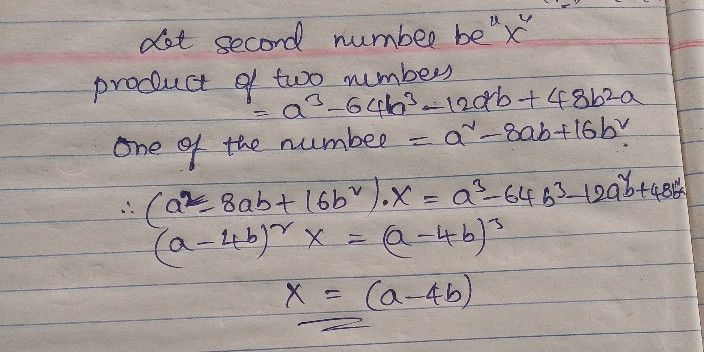Symbol
ProblemThe product of two numbers is $\left(a^{3}-64b^{3}-12a^{2}b+48ab^{2}\right)$ If one of the numbers is $1$ $\left(8^{2}$ $8ab+16b^{2}\right)$ find the other number. E $=O∠$ $\square$